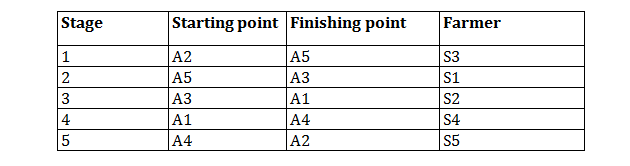Directions for questions no. (1-5): A circular field, with inner radius of 10 meters and outer radius of 20 meters, was divided into five successive stages for ploughing. The ploughing of each stages was handed over to a different farmer.
1. Farmers are referred to by following symbols: S1, S2, S3, S4, S5.
2. The points between different stages of project are referred to by the following symbols: A1, A2, A3, A4, A5, not necessarily in the same order.
3. Farmer S4 was given the work of the fourth stage.
4. Stage 3 finished at point A1, and the work of which was not given to farmer S1.
5. Farmer S3 was given work of stage ending at point A5.
6. Farmer S5 was given the work of ploughing stage starting at point A4.
7. The stage from point A5 to point A3 was not the first stage.

Question 1: Which were the starting and the finish points of stage 2?
A. A2 and A5
B. A5 and A3
C. A3 and A1
D. A5 and A4
E. A3 and A2

Question 2: For which farmer was A2 a finishing point?
A. S1
B. S2
C. S3
D. S4
E. S5

Question 3: Which stage was ploughed by farmer S5?
A. First
B. Second
C. Third
D. Fourth
E. Fifth

Question 4: Which was the starting point for Farmer S3?
A. A2
B. A3
C. A4
D. A1
E. None of above

Question 5: Which was the finish point for farmer S2?
A. A1
B. A2
C. A3
D. A4
E. A5

Solution:

For questions 1 to 5: Stage 3 finished at point A1 and the work in the stage 4 was done by S4. It is given that one of the stage starts with A5 and ends with A3, and that stage cannot be the stage 1 (Condition 4), stage 3 (Condition 6), stage 4 (Condition 6) or stage 5 (Condition 4). Hence, stage 2 starts and ends with point A5 and A3 respectively. Therefore, S3 has ploughed in stage 1 (Condition 7). So stage 5 will start and end with A4 and A2 respectively (Condition 3) So the work of stage 2 and stage 3 will be done by S1 and S2 respectively(Condition 6). Based on the inferences, the following table can be formed: